# Solving Linear Equations MultiStep Equations Solving MultiStep Equations

• Slides: 15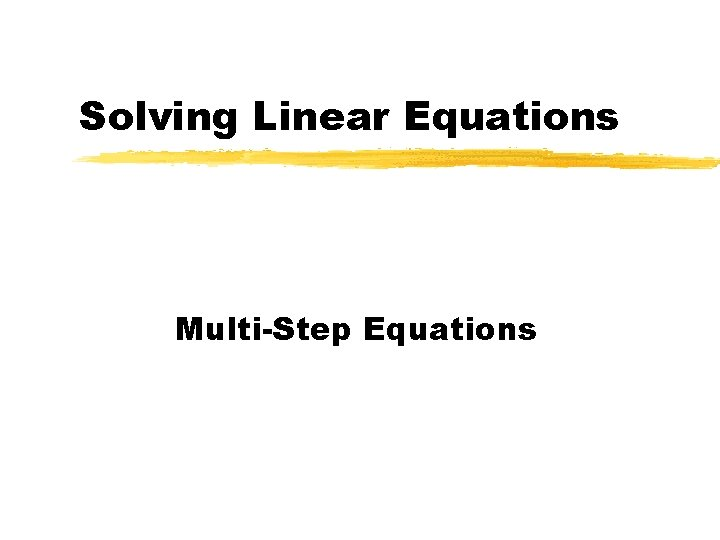Solving Linear Equations Multi-Step EquationsSolving Multi-Step Equations z. To find the solution to an equation we must isolate the variable. z. We isolate the variable by performing operations that will eliminate (cancel) the other numbers from the expression.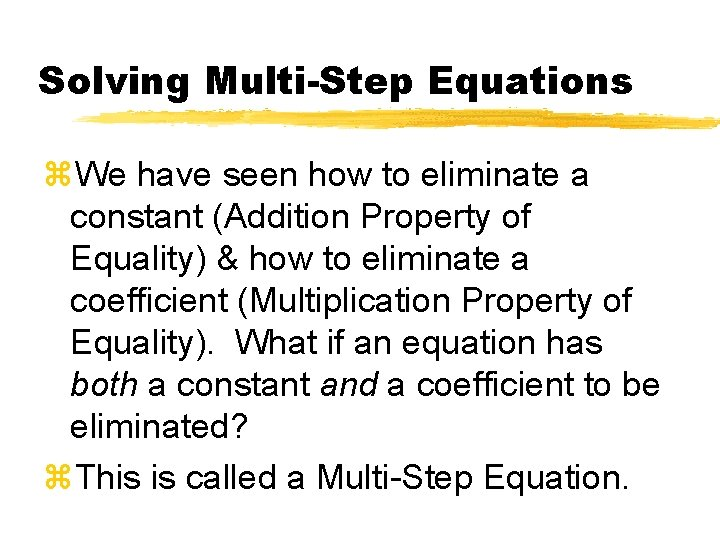Solving Multi-Step Equations z. We have seen how to eliminate a constant (Addition Property of Equality) & how to eliminate a coefficient (Multiplication Property of Equality). What if an equation has both a constant and a coefficient to be eliminated? z. This is called a Multi-Step Equation.Solving Multi-Step Equations z. This is a multi-step equation. 3 x + 5 = 17 z. We must eliminate the constant, 5, and the coefficient, 3, to isolate the variable, x. z. The order that we use the equality properties is very important, we must follow the order of operations in reverse!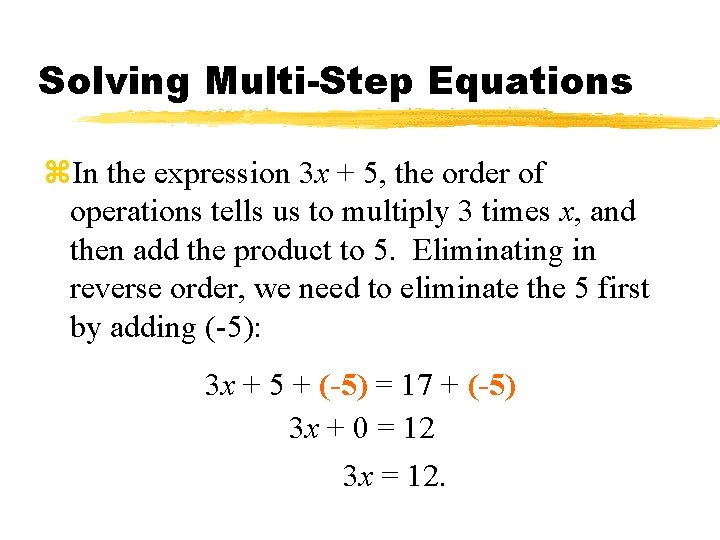Solving Multi-Step Equations z. In the expression 3 x + 5, the order of operations tells us to multiply 3 times x, and then add the product to 5. Eliminating in reverse order, we need to eliminate the 5 first by adding (-5): 3 x + 5 + (-5) = 17 + (-5) 3 x + 0 = 12 3 x = 12.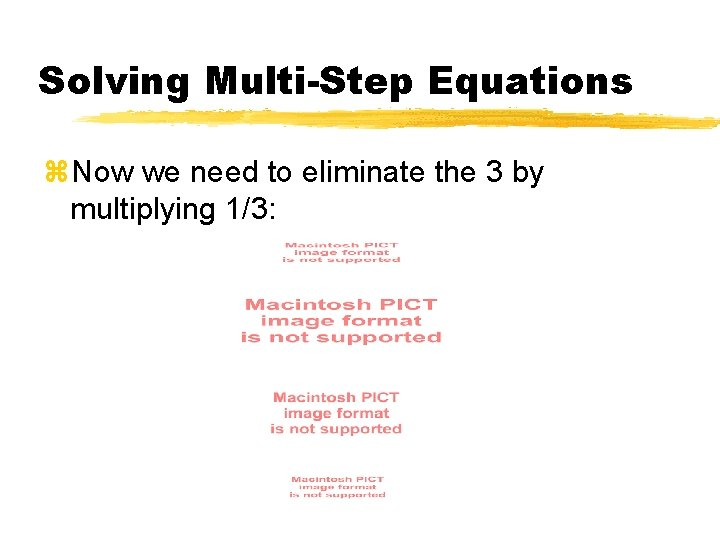Solving Multi-Step Equations z. Now we need to eliminate the 3 by multiplying 1/3:Solving Multi-Step Equations z. Check the solution: 3 x + 5 = 17 Replace x with 4. Multiply. Add. 3(4) + 5 = 17 12 + 5 = 17 17 = 17. √Solving Multi-Step Equations z. The Steps: y. If the variable is on the right side of the equation, swap sides. y. If there is a constant on the variable side, add the opposite of the constant to both sides of the equation. y. If there is a coefficient in front of the variable, multiply both sides of the equation by the reciprocal of the coefficient.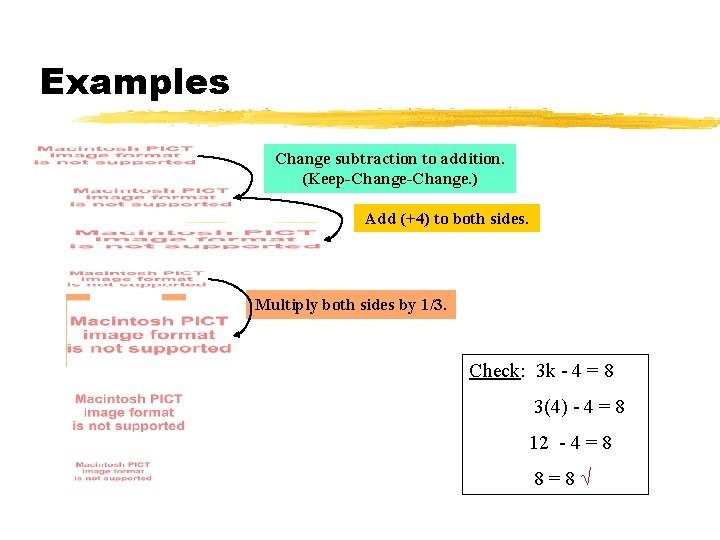Examples Change subtraction to addition. (Keep-Change. ) Add (+4) to both sides. Multiply both sides by 1/3. Check: 3 k - 4 = 8 3(4) - 4 = 8 12 - 4 = 8 8=8√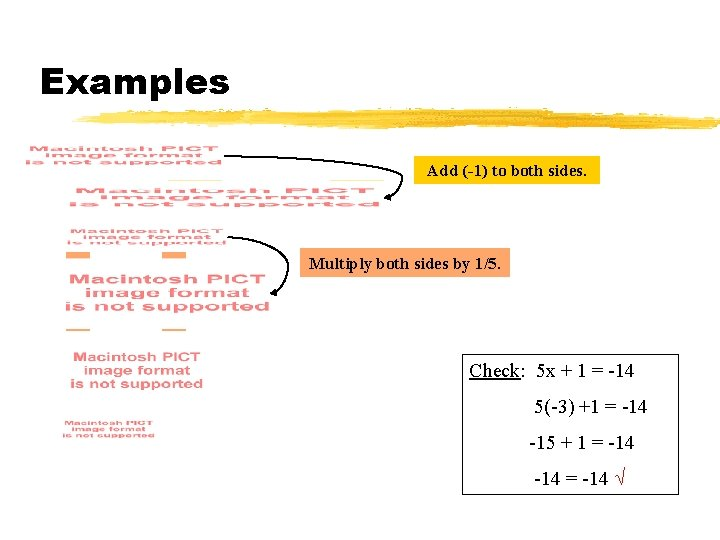Examples Add (-1) to both sides. Multiply both sides by 1/5. Check: 5 x + 1 = -14 5(-3) +1 = -14 -15 + 1 = -14 √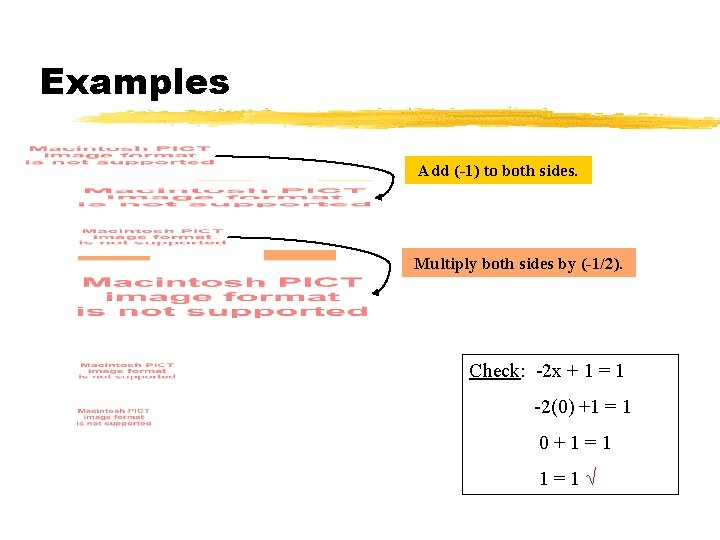Examples Add (-1) to both sides. Multiply both sides by (-1/2). Check: -2 x + 1 = 1 -2(0) +1 = 1 0+1=1 1=1√Try These!Solutions!Solutions!Solutions!